International
Tables for
Crystallography
Volume C
Mathematical, physical and chemical tables
Edited by E. Prince

International Tables for Crystallography (2006). Vol. C, ch. 6.4, p. 610

## Section 6.4.5. Primary extinction

T. M. Sabinea

aANSTO, Private Mail Bag 1, Menai, NSW 2234, Australia

### 6.4.5. Primary extinction

| top | pdf |

Zachariasen (1967) introduced the concept of using the kinematic result in the small-crystal limit for σ, while Sabine (1985, 1988) showed that only the Lorentzian or Fresnellian forms of the small crystal intensity distribution are appropriate for calculations of the energy flow in the case of primary extinction. Thus,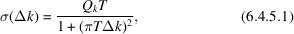where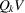is the kinematic integrated intensity on the k scale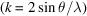,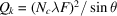, and T is the volume average of the thickness of the crystal normal to the diffracting plane (Wilson, 1949). To include absorption effects, which modify the diffraction profile of the small crystal, it is necessary to replace T by TC, where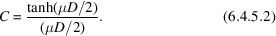To determine the extinction factor, E, the explicit expression for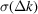[equation (6.4.5.1)] is inserted into equations (6.4.4.3)and (6.4.4.4), and integration is carried out over Δk. The limits of integration are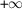and. The notation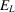and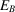is used for the extinction factors at 2θ = 0 and 2θ = π rad, respectively.

After integration and division by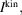it is found that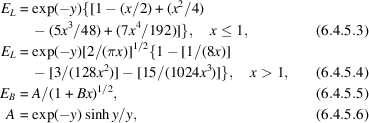and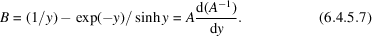In these equations,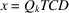and y = μD.

### References

Sabine, T. M. (1985). Extinction in polycrystalline materials. Aust. J. Phys. 38, 507–518.
Sabine, T. M. (1988). A reconciliation of extinction theories. Acta Cryst. A44, 368–373.
Wilson, A. J. C. (1949). X-ray optics. London: Methuen.
Zachariasen, W. H. (1967). A general theory of X-ray diffraction in crystals. Acta Cryst. 23, 558–564.